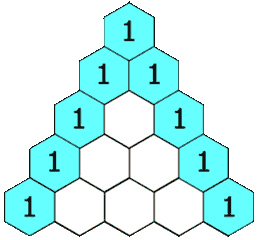By Long Luo

# Intuition

To generate a Pascal’s triangle is as the below animated git shows.We can find that the Current Layer has only one element more than the Previous Layer. The elements in the Current Layer are equal to the elements in the Previous Layer, which was added one by one after being shifted one bit backward.

Therefore, we only need to deal with the Last Layer. We add a $0$ at the beginning and the end of the Last Layer. Then sum the corresponding positions to get a New Layer.

## Analysis

• Time Complexity: $O(n^2)$.
• Space Complexity: $O(1)$.

All suggestions are welcome.
If you have any query or suggestion please comment below.
Please upvote👍 if you like💗 it. Thank you:-)

Explore More Leetcode Solutions. 😉😃💗